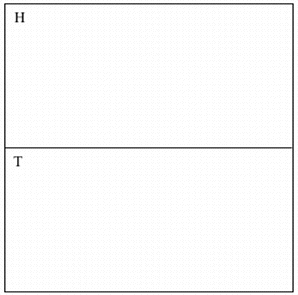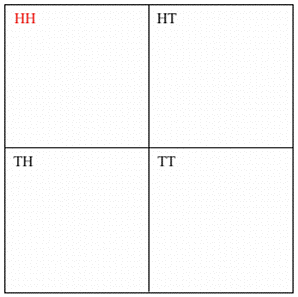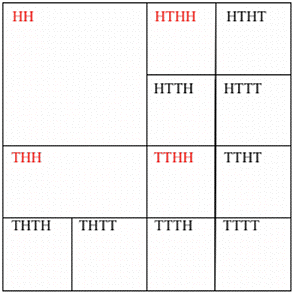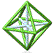PCMI, Summer 2004
High School Teacher Program

July 14: Consecutive Coin Flips by Connie Savoie

Flipping to Win

The following is an example of using an area model to solve a probability problem.

The Problem:
In a coin flipping game, you flip a fair coin ten times. If you flip heads twice in a row at any point during the game, you lose. Find the probability of winning.

Process:
I used an area model in order to find the solution. The process is as follows.

1. Draw a square, 32 x 32 units in dimension. (There are 210, or 1024, outcomes. (1024)_ =32, so a 32 x 32 unit square gives 1024 boxes)
2. Divide the square in half, representing the first coin flip.3. Divide each rectangle in half again, representing the second coin flip.4. Continue dividing each section to represent the 10 coin flips. Stop dividing the sections any time two heads are flipped in a row since two heads is a ŇloseÓ and it makes no sense to continue. The next two flips (four rounds total) are shown below:5. Solution:
Upon completion of the area model, I counted the "winning" boxes. There were 144 possible wins out of 1024 total possible outcomes, for a winning probablity of 144/1024 = 9/64, or about 14%.

This method isn't exactly elegant and is definitely a bit time consuming, but an interesting fractal-type pattern emerges. It's also very accessable for students. I use the area model extensively with my freshmen in Interactive Math (IMP) 1.

I did the original model by hand on graph paper and redid it in Microsoft Excel. The complete area model shows the winning boxes as unshaded. The numbers on the right side of the area model are simply where I counted up the winning boxes.

Cheers!
Connie Savoie
West Seattle High School
PCMI, HSTP

Unless otherwise indicated all of these materials are licensed under a© 2001 - 2020 Park City Mathematics Institute IAS/Park City Mathematics Institute is an outreach program of the Institute for Advanced Study, 1 Einstein Drive, Princeton, NJ 08540. Send questions or comments to: Suzanne Alejandre